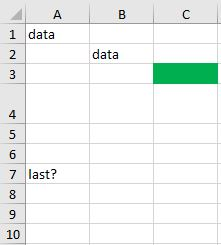### Get started with Spring 5 and Spring Boot 2, through the Learn Spring course:

>> CHECK OUT THE COURSE

## 1. Overview

In this tutorial, we’ll discuss how to find the last row in an Excel spreadsheet using Java and Apache POI.

Firstly, we’ll see how to fetch a single row from the file using Apache POI. Then, we’ll look at methods for counting all rows in a worksheet. Finally, we’ll combine them to fetch the last row of a given sheet.

## 2. Fetch a Single Row

As we already know, the Apache POI provides an abstract layer to represent Microsoft documents, including Excel, in Java. We can access the sheets in a file and even read and modify each cell.

Let’s start by fetching a single row from our Excel file. Before we move on, we need to get the Worksheet from the file:

``````Workbook workbook = new XSSFWorkbook(fileLocation);
Sheet sheet = workbook.getSheetAt(0);``````

The Workbook is a Java representation of the Excel file, while Sheet is the main structure within a Workbook. The Worksheet is the most common subtype of Sheet, representing a grid of cells.

When we open our worksheet in Java, we can access the data it contains, i.e., the row data. To fetch a single row, we can use the getRow(int) method:

``Row row = sheet.getRow(2);``

The method returns the Row object – the high-level representation of a single row from the Excel file, or null if the row doesn’t exist.

As we see, we need to supply a single parameter, the index (0-based) of the requested row. Unfortunately, there is no API available to get the last row directly.

## 3. Find the Count of Rows

We’ve just learned how to get a single row from an Excel file using Java. Now, let’s find the index of the last row on a given Sheet.

Apache POI provides two methods that help count rows: getLastRowNum() and getPhysicalNumberOfRows(). Let’s take a look at each of them.

### 3.1. Using getLastRowNum()

According to the documentation, the getLastRowNum() method returns the number (0-based) of the last initialized row on the worksheet, or -1 if no row exists:

``int lastRowNum = sheet.getLastRowNum();``

Once we fetched lastRowNum, we should now easily access the last row using the getRow() method.

We should note that rows that had content before and were set to empty later might still be counted as rows. Therefore, the result may not be as expected. To understand this, we need to learn more about physical rows.

### 3.2. Using getPhysicalNumberOfRows()

Inspecting the Apache POI documentation, we can find a special term related to the rows – the physical row.

A row is always interpreted as physical whenever it contains any data. The row is initialized not only if any cells in that row contain text or formulas but also if they have some data about formatting, e.g., the background color, the row height, or non-default font used. In other words, each row that is initialized is also physical.

To get the count of physical rows, Apache POI provides the getPhysicalNumberOfRows() method:

`int physicalRows = sheet.getPhysicalNumberOfRows();`

According to the physical row explanation, the result may differ from the number obtained with the getLastRowNum() method.

## 4. Fetch the Last Row

Now, let’s test both methods against a more complex Excel grid:Here, the leading rows contain the text data, the value calculated by the formula (=A1), and the background color changed accordingly. Then, the 4th row has modified height, while the 5th and 6th rows are untouched. The 7th row contains text again. On the 8th row, the text was previously formatted but later cleared. The 9th and subsequent rows weren’t edited.

Let’s check the results of the count methods:

``````assertEquals(7, sheet.getLastRowNum());
assertEquals(6, sheet.getPhysicalNumberOfRows());``````

As we mentioned before, the last row number and the physical number of rows are different in some cases.

Let’s now fetch rows based on their index:

``````assertNotNull(sheet.getRow(0)); // data
assertNotNull(sheet.getRow(1)); // formula
assertNotNull(sheet.getRow(2)); // green
assertNotNull(sheet.getRow(3)); // height
assertNull(sheet.getRow(4));
assertNull(sheet.getRow(5));
assertNotNull(sheet.getRow(6)); // last?
assertNotNull(sheet.getRow(7)); // cleared later
assertNull(sheet.getRow(8));
...``````

As we can see, the getPhysicalNumberOfRows() returns the total number of not-null (i.e., initialized) Rows in the worksheet. The getLastRowNum() value is the index of the last not-null Row.

Therefore, we can fetch the last row on the sheet:

``````Row lastRow = null;
int lastRowNum = sheet.getLastRowNum();
if (lastRowNum >= 0) {
lastRow = sheet.getRow(lastRowNum);
}``````

However, we have to remember that the last row returned by Apache POI is not always the one where text or formula is shown, especially in some UI editors such as Microsoft Excel.

## 5. Conclusion

In this article, we inspected the Apache POI API and fetched the last row from a given Excel file.

We started by revisiting some of the basic methods to open a spreadsheet in Java. We then introduced the getRow(int) method to retrieve a single Row. After that, we checked the values of getLastRowNum() and getPhysicalNumberOfRows() and explained their difference. Finally, we checked all the methods against an Excel grid to fetch the last row.

As always, the full version of the code is available over on GitHub.

### Get started with Spring 5 and Spring Boot 2, through the Learn Spring course:

>> CHECK OUT THE COURSE